10 Breaking the Coulomb barrier by electrostatic acceleration of positive ions towards electrons moving in a circle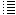Just some idea's...

(10e idea)

The goal is to design a kind of fusor ( http://en.wikipedia.org/wiki/Fusor ). But instead of a fixed inner grid, which causes losses, we try to create a kind of negative potential well by injecting electrons inside the sphere.

In the polywell also electrons are injected. Here several magnets try to keep the electrons confined inside the sphere.

Let us now consider the following device with only two magnets:

Fig. 1. Schematic representation of an electrostatic magnetic fusion device.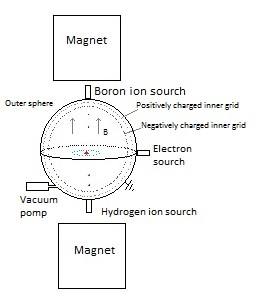The (outer) sphere has been pumped vacuum and is earthed.

Above and under the sphere a magnet is placed which generates an (approximately) uniform (vertical) magnetic field inside the sphere.

With an electron source (electron gun) we shoot electrons in a horizontal plane inside the sphere.

Because of the magnetic field the electrons will move in circles.

Fig. 2. Circular movement of electrons in an uniform magnetic fieldIn an electron gun the electrons are accelerated till about 30 keV
( http://nl.wikipedia.org/wiki/Elektronenkanon )

½ m.v=  30.103.1,6.10-19 J   =>   v = (2.30.103.1,6.10-19 / (9,11.10-31 ))  =  1,0.108 m/s ( speed of light is 3,0.108 m/s)

If we want the electrons to circulate in an orbit with a radius of, for example, r = 2 cm, then we need a magnetic field of:

B = m.v / (r.q) = 9,11.10-31.1,0.108 / (0.02.1,6.10-19 ) = 2,8. 10-2 T = 280 gauss  (actually we should also have taken into account relativistic effects...)

This is still less than a loudspeaker magnet, so we do not need strong magnets.  (http://en.wikipedia.org/wiki/Orders_of_magnitude_(magnetic_field) )

But I don't think it's necessary that the electrons have such a high speed. Let's take 100 eV.

v = (2.100.1,6.10-19 / (9,11.10-31 ) )  =  5.93. 106 m/s  (this is way lower than the speed of light, so no relativistic effects)
B = m.v / (r.q) = 9,11.10-31.5.93. 106  / (0.02.1,6.10-19 ) = 1.7. 10-3 T = 17 gauss

Several values:

 radius orbit electrons r1 energy electrons eV speed electrons magnetic field 2,0 cm 30 keV 1,0.108 m/s 280 gauss 2,0 cm 1 keV 1,9.107 m/s 54 gauss 2,0 cm 200 eV 8,4.106  m/s 24 gauss 2,0 cm 100 eV 5.93.106 m/s 17 gauss

The classical formula for the radiated power from an accelerated electron is

(1)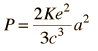Let's calculate this for electrons with 100 eV:

K= 8.987.10Nm2/C2 ;  c = 3.108 m/s ; e = 1,6.10-19 C

a = v2/r =  (5.93. 106)2  / 0.02 =  1.76.1015   m/s2

->  P = 2 . 8.987.109 . (1,6.10-19 )2. / (3.(3.108)3)  .   (1.76.1015 )2 =  1.76  .10-23  J/s  (for 1 electron)

This will cause  a  decrease in kinetic energy of an electron in 1 s: Δ ½mv2 = 1.76  .10-23 J
->
Δ v = ( 2. 1.76  .10-23/(9,11.10-31)  ) = 6.2.103   m/s  ->  Δ v = 6.2 . 103 / 5.93.106  x 100 % = 0.1. % in 1 second.

How many electrons produces an electron gun?

A Nanocoulombmeter in combination with a Faraday cup can be used to detect and measure the beams emitted from electron guns and ion guns. ( http://en.wikipedia.org/wiki/Nanocoulombmeter

In the link under an electron gun is sold with an energy range: 1 eV to 100 keV and a beam current range: 1 nA to 20 mA
( http://www.kimballphysics.com/electron-gun-systems/product-overview )

1.10-9 A = 1.10-9 C/s ~ 1.10-9 C/s / (1,6.10-19 C) = 6,3. 109   electrons/s

It seems therefore plausible to have an electron gun that produces about 1. 109    electrons/ sec.

The sphere we give a radius of 25 cm.

After 1 s there are 109   electrons inside the sphere and for the voltage calculation we assume that they are located in the centre of the sphere.

Fig. 3. Horizontal cross section with a potential difference ΔV  caused by the accumulation of the electronsr1 = the radius of the circular movement of the electrons;
r2= the radius of the sphere;
Q = the total charge of all electrons that are moving in a circular orbit inside the sphere;
ΔV = the voltage difference between the position of the electrons and the sphere;

After 1 s we have Q = 109 . 1,6.10-19 =  1,6.10-10 C  (if no losses; this is like a current of 1,6.10-10 A)

r1 = 2,0 cm;   r2 = 25 cm

1/(4π є0)= 9.109  (Nm2/C2)

ΔV = - 1,6.  10-10 .  9 . 109 ( 1/0.02 - 1/0,25)  = - 66,24 V  (C.Nm2/(C2 . m) = Nm/C)

After one hour: ΔV = 3600 . - 66,24 V = -2,4 . 105 V

And after  4 hours: ΔV = 4. -2,4 . 105 =  - 9.6  . 105 V  ≈ - 1 MV

Will the electrons stay in orbit? How many electrons will escape?

Fig. 4. Positive charged boron ions and hydrogen ions are shot into the sphere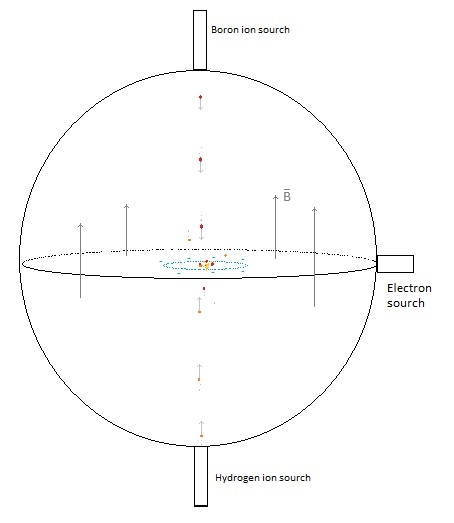The positive ions move first parallel to the magnetic field, so they will no feel any Lorentz force and will keep moving in a straight line.

The accumulation of electrons in the centre causes a negative potential well and the positive boron and hydrogen ions will be accelerated towards the centre.

The moving electrons generate also a magnetic field that will exert a Lorentz force on the moving positive ions.  We will assume (can this be done?) that the boron and hydrogen ions still reach the centre area.

Some of the ions will collide and others continue moving straight ahead after which they will be slowed down because of the negative potential well and move back again towards the centre area.

Some ions will obtain a higher speed after a collision, and some of these ions collide again and some of them obtain an even higher speed, and collide again and obtain an even higher speed etc.  And sometimes two ions, a boron and a hydrogen, with a very high speed collide and fuse in the boron-hydrogen fusion reaction:

115B + 11H  -> 3 42He  + 8,68 MeV   (see en.wikipedia.org/wiki/Aneutronic_fusion)

No noxious neutrons are produced and the 3 positive  42He+ particles will move with high speed out of the centre increasing the voltage.

Inside the sphere, we place two grids. The first grid with a diameter of 22 cm we charge negatively with a potential of (for example) -9 MeV, and the second grid with a diameter of 24 cm is  (for example) + 100 V positively charged.

Fig. 5. The sphere with two grids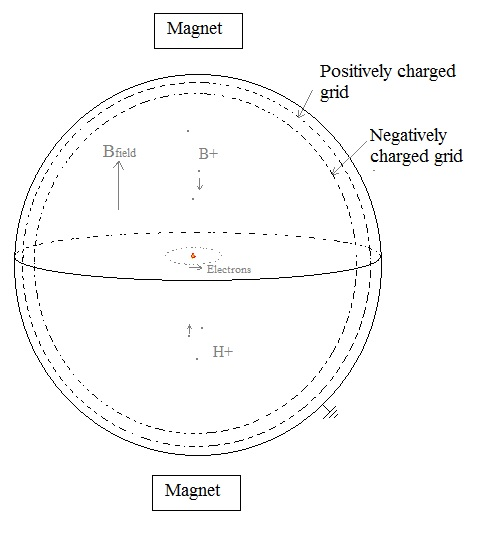With these two grids and the applied voltage we try to avoid that any high energy boron or hydrogen particles escape, and we stop the 42He2+ particles transferring their kinetic energy into electric energy (after fusion they will escape with 8.68 MeV).

But the incoming positive ions of boron and hydrogen should not go through the voltage difference between the two grids. They would increase speed, which would be positive, but the invested energy is difficult to recover..  Perhaps we let them travel through a conducting pipe the first part?  No, because ʃ E.dl = 0    What we could do is first let in some ions and than apply a voltage to the grid.

Some ions could collide with the grid and this would cause energy looses. How much??

Computer program:

Computer simulation

 (Jan. 2013) (Jan. 2013) (Jan. 2013) (3 Febr. 2013) (6 Febr. 2013) (8 Febr. 2013) (16 Jan. 2014) (17 Jan. 2014) (20 Jan. 2014) ( Febr. 2014- 2016) Computer simulation tenth idea (2014-2016) Eleventh idea   (2016.. 2017)20  February 2014 - 2016      by  Rinze Joustra        www.valgetal.com